# HBSE 9th Class Maths Notes Chapter 5 Introduction to Euclid’s Geometry

Haryana State Board HBSE 9th Class Maths Notes Chapter 5 Introduction to Euclid’s Geometry Notes.

## Haryana Board 9th Class Maths Notes Chapter 5 Introduction to Euclid’s Geometry

Introduction
The word ‘geometry’ is derived from the Greek words ‘geo’, means the ‘earth’, and ‘metrein’, means ‘to measure’. So, it appears to have originated from the need for measuring land. Many ancient civilization of Egypt, Babylonia, India, Greece, China have studied the ‘geometry’ in various forms. They short out the several practical problems which required the development of geometry in various ways.

In Indus Valley Civilization, the ratio length : breadth : thickness of the bricks was taken as 4 : 2 : 1.

Construction of altars (or vedis) and fireplaces for performing Vedic rites were originated in Vedic period. Square and circular altars were used for household rituals, while shape of altars were the combination of rectangles, triangles and trapeziums. They are used for public worship. The ‘sriyantra’ consists of nine interwoven isosceles triangles which are arranged in such a way that they produce 43 subsidiary triangles. A Greek mathematician, Thales, (640 BC) gave the first known proof of the statement that a circle is bisected (cut into two equal parts) by its diameter.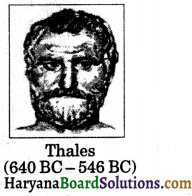Pythagoras (572 BC) was the most famous pupil of Thales discovered many geometric properties and developed the theory of geometry to a great extent. This process continued till 300 BC. His important theorem is; In right triangle, the square of hypotenuse is equal the sum of the square of base and square of perpendicular.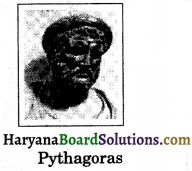Euclid (300 BC) was a mathematics teacher who was born in Alexandria in Greece introduced the method of proving mathematical results by using deductive logical reasoning and the previously proved results. The geometry of plane figure is known as “Euclidean Geomery.”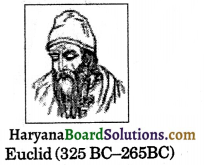The Indian Mathematician, Aryabhatta (born 476 AD) worked out the area of an isosceles triangle, the volume of a pyramid and approximate value of π.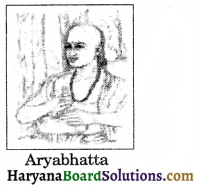Brahmagupta (born 598 AD) discovered the formula for finding the area of cyclic quadrilateral.Bhaskara II (born 1114 AD) gave a dissection proof of Pythagoras’ theorem.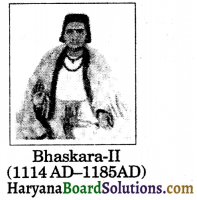In this chapter, we shall study the Euclid’s approach to geometry.

Key Words
→ Version – Translation.

→ Altars – Raised plateform used for sacrifice or religious offering.

→ Rituals – Way of performing religious services.

→ Rite – Religious ceremony.

→ Subsidiary – Accessory.

→ Allied – Related.

→ Extent – Large space or tract size.

→ Volume – Space occupied, mass.→ Cyclic Quadrilateral – A quadrilateral whose four vertices lie on a circle.

→ Proof – The course of reasoning which establishes the truth of a statement.

→ Logic – The science or art of reasoning.

→ Physical – Belonging to physics.

→ Universal – Belonging to all.

→ Fact – A thing known to be true.

→ Coincide – To occupy the same space.

→ Proposition – A statement of something to be done.

→ Interpreted – To exposed the meaning of.

→ Demonstrate – To prove with certainly.

→ Consequence – result.

→ Superposed – To put or place over or above.

→ Assumption – A supposition, thing assumed.Basic Concepts
Euclid’s Definitions, Axioms and Postulates:
(a) Euclid summarised the work of ‘geometry’ as definitions. He listed 23 definitions in Book 1 of ‘Elements’. A few of them are given below:

• A point is that which has no part.
• A line is breadthless length.
• The ends of a line are points.
• A straight line is a line which lies evenly with the points on itself.
• A surface is that which has length and breadth only.
• The edges of surface are lines.
• A plane surface is a surface which lies evenly with the straight lines on itself.

In these definitions, we observe that, point, line, breadth, length, plane etc. are undefined terms. The only thing is that we can represent them intuitively or explain them with the help of ‘physical models.’

Starting with these definitions, Euclid assumed certain properties, which were not to be proved. These assumptions are actually “obvious universal truths”. He divided them into two types: axioms and postulates. He used term ‘postulate’ for the assumptions that were specific to geometry. Common notions (often called axioms), on the other hand, were. assumption used throughout mathematics and not specifically linked to geometry.

(b) Axioms: The basic facts which are taken for granted, without proof, are called axioms.
Some of the Euclid’s axioms (not in order) are given below:
(i) Things which are equal to the same thing are equal to one another.
i.e., If p = q and r = q, then p = r.
(ii) If equals are added to equals, the wholes are equal.
i.e., If p = q, then p + r = q + r.
(iii) If equals are subtracted from equals, the remainders are equal.
i.e., If p = q, then p – r = q – r.
(iv) Things which coincide with one. another are equal to one another.
(v) The whole is greater than the part ie., If p>q, then there exists r(r ≠ 0) such that
P = q + r.
(vi) Things which are double of the same things are equal to one another. i.e., If p = q, then 2p = 2q.
(vii) Things which are halves of the same things are equal to one another.
Le. If p = q, then $$\frac{p}{2}=\frac{q}{2}$$

(c) Postulate: A statement whose validity is accepted without proof, is called a postulate.
Euclid gave five postulates stated as below:
Postulate 1: A straight line may be drawn from any one point to any other point.
This postulate tells us that at least one straight line passes through two distinct points which is shown in the figure below: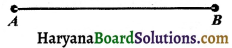Axiom 5.1. Given two distinct points, there is a unique line that passes through them.

In the given figure, P and Q are two distinct points. Out of all lines passing through the point P, there is exactly one line ‘l’ which also passes through Q. Also out of all lines passing through the point Q, there is exactly one line l which also passes through the point P. Hence, we find there is a unique line l which passes through the points P and Q.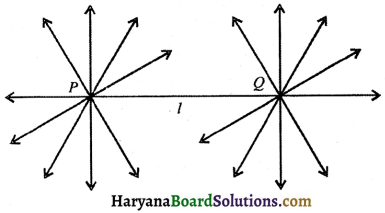Postulate 2: A terminated line can be produced indefinitely.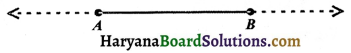Postulate 3: A circle can be drawn with any centre and any radius.
Postulate 4: All right angles are equal to one another.
Postulate 5: If a straight line falling on two straight lines makes the interior angles on the same side of it taken together less than two right angles, then the two straight lines, if produced indefinitely, meet on that side on which the sum of angles is less than two right angles.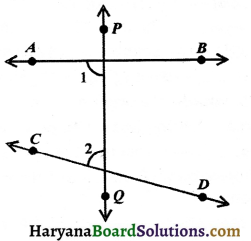For example, in the figure line PQ falls on the lines AB and CD such that ∠1 + ∠2 < 180° on the left side of PQ. Therefore, the lines AB and CD will eventually intersect on the left side of PQ.

(d) Statement: A sentence which can be judged either true or false is called a statement.
For example:
(i) The sum of the angles to a quadrilateral is 360°, is a true statement.
(ii) The sum of the angles of a triangle is 90°, is a false statement.
(iii) y + 5 > 9 is a sentence but not a statement.Theorem: In mathematics, a theorem is a statement that has been proven on the basis of previously established statements, such as other theorems and previously accepted statements, such as axioms. The derivation of a theorem is often interpreted as a proof of the truth of the resulting expression, but different deductive systems can yield other interpretations, depending on the meanings of the derivation rules. The proof of a mathematical theorem is logical argument demonstrating that the conclusion.

For example:
(i) The area of a triangle is equal to half its base, multiplied by its attitude.
(ii) An exterior angle of a triangle is equal to sum of its opposite interior angles.
Corollary: A proposition, whose truth can easily be deducted from a preceeding theorem, is called its corollary.

(e) Some terms allied to Geometry:
(i) Point: A point is a mark of position, which has no length, no breadth and no thickness. We represent a point by a capital letters A, B, C, P, Q etc., as shown in the figure.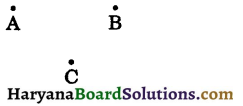(ii) Plane: A plane is a every surface such that point of the line joining any two points on it, lies on it.
The surface of the top of the table, surface of the smooth blackboard and surface of a sheet of paper are the some close examples of a plane surfaces are limited in extent but geometrical plane extends endlessly in all directions.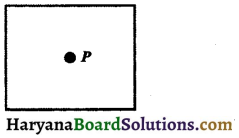(iii) Line: A line has length but no breadth or thickness. A line has no ends points. A line has no definite length. It can be extended infinitely in both the directions. The given figure shows line $$\bar{AB}$$.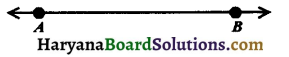Some times we shall denote lines by small letters such as l, m, n, p etc.

(iv) Ray: It is a straight line which starts from a fixed point and moves in the same direction. A ray has one endpoint and it has no definite length.
The given figure shows a ray $$\overrightarrow{A B}$$.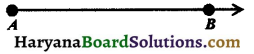(v) Line segment: It is a straight line with its both ends fixed. The given figure shows a line segment $$\bar{AB}$$, with fixed ends A and B. A line has a definite length.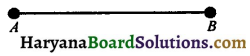So, a line segment is the shortest distance between two fixed points.

(vi) Collinear points: Three or more than three points are said to be collinear, if they lie on the straight line.
The given figure shows the collinear points P, Q, R while A, B, C are non-collinear.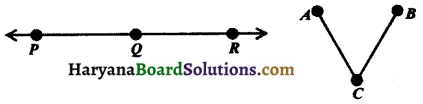(vii) Parallel lines: The straight lines which lie in the same plane and do not meet at any point on producing on either side, are called parallel lines.
If l and m are parallel lines in a plane, we write l || m.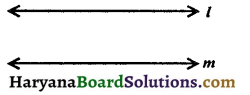The distance between the two parallel lines always remains same.

(viii) Intersecting lines: Two lines having a common point are known as intersecting lines.
The common point (O, in figure 5.18) is known as the point of intersection.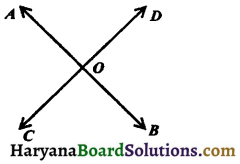(ix) Concurrent lines: In the figure 5.19, the lines pass through the same point. In this case, lines are called concurrent lines.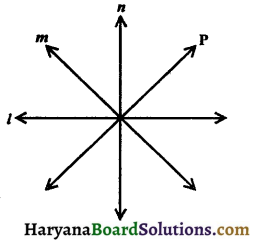(x) Congruence of line segment: If the given two line segments are of the same size, they are said to be ‘congruent. Two lines AB and CD are congruent, if the trace copy of one can be superposed on the other so as to cover it completely and exactly.
Symbolically, $$\bar{AB}$$ ≅ $$\bar{CD}$$ or line segment AB ≅ line segment CD.

Congruence relation in the set of all line segments:
(i) AB ≅ AB
(ii) AB ≅ CD ⇒ CD ≅ AB
(iii) AB ≅ CD and CD ≅ EF, then AB ≅ EF.Theorem 5.1.
Two distinct lines cannot have more than one point in common.
Proof. Here, we are given two lines I and m. We need to prove that they have only one point in common. If possible, let P and Q two points common to the given lines l and m. Then the line I passes through the points P and Q and line m passes through the points P and Q. But this assumption clashes with the axiom that one and only one line can pass through two distinct points. So, our supposition that two lines pass through two distinct points is wrong.
Hence, two distinct lines cannot have more than one point in common.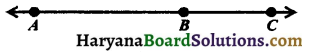Equivalent Versions of Euclid’s Fifth Postulate:
There are several equivalent versions of fifth postulate of Euclid. One of them is ‘Playfair’s Axiom’ (given by Scottish mathematician John Playfair in 1729), as stated below:

Playfair’s Axiom: “For every line l and for every point P not lying on l, there exists a unique line m passing through P and parallel to l.”
From figure 5.52, we observe that all the lines passing through the point P, only line m is parallel to line l.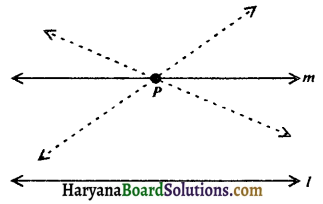This result can also be stated as: Two distinct intersecting lines cannot be parallel to the same line.